Cats

Two cats caught two mice in two days. How many mouses will catch 6 cats for 6 days?

Result

x =  18

Solution:Leave us a comment of this math problem and its solution (i.e. if it is still somewhat unclear...):Be the first to comment!Next similar math problems:

1. Chickens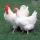2 chickens give 2 eggs in 2 days. How many eggs can give 8 chickens for 8 days?
2. Foot in busIt was 102 people on the bus. 28 girls had two dogs. A 11 girls had one dog. At the next stop seceded 5 dogs (even with their owners). They got two boys together with three dogs. The bus drove one driver. How many foot were in bus?
3. Pins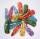Sarah bought 9 pins, Eva bought 4 pins and saved 2 euros. How many pins buy Dana, when she have 3 euro?
4. Trio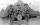56 children lined up in groups of three. How many children did not create a trio?
5. The resultHow many times I decrease the number 1632 to get the result 24?
6. DoctorsIn the city operates 171 doctors. The city has 128934 citizens. How many citizens are per one doctor?
7. Math classificationIn 3A class are 27 students. One-third got a B in math and the rest got A. How many students received a B in math?
8. Train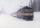The train passes part of the line for 95 minutes at speed 75 km/h. What speed would have to go in order to shorten the driving time of 20 minutes?
9. School trip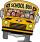School trip cost 247.2 Eur for one class (24 students). How much would cost a trip for two classes? (both classes together have 53 students)
10. CagesHonza had three cages (black, silver, gold) and three animals (guinea pig, rat and puppy). There was one animal in each cage. The golden cage stood to the left of the black cage. The silver cage stood on the right of the guinea pig cage. The rat was in the
11. Wire cut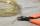A wire of length 7 m was cut into equal lengths using 4 cuts. How long is each piece?
12. Unknown number 16My number's tens is three times more then ones My number's ones is twice the number of thousands and my number's hundreds is half the number of of tens. I have two ones. which number am I?
13. The largest numberFind the largest integer such that: 1. No figures is not repeat, 2. multiplication of every two digits is odd, 3. addition all digits is odd.
14. ProductResult of the product of the numbers 1, 2, 3, 1, 2, 0 is:
15. Addition of Roman numbersAdded together and write as decimal number: LXVII + MLXIV
16. Expression plus minusEvaluate expression: (-1)2 . 12 – 6 : 3 + (-3) . (-2) + 22 – (-3) . 2
17. NumberCalculate the integer number which, divided by 34 gives 10 and the rest 25.